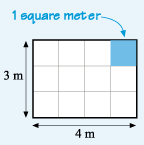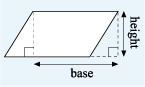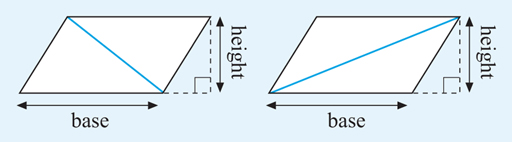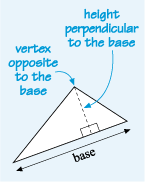Succeed with maths – Part 2

Start this free course now. Just create an account and sign in. Enrol and complete the course for a free statement of participation or digital badge if available.

Free course

# 3.1 Formulas for areas

Many areas can be built out of basic shapes such as rectangles, triangles or circles, and these areas can be calculated using formulas. For example, if a rectangular room measures 4 m by 3 m and you are covering it in carpet tiles that are each 1 m square, the tiles can be arranged in three rows, each with four tiles. So the total number of tiles will be 3 x 4 = 12 and the area covered is written as 12 m2.Figure 19 A rectangle

This area could have been calculated directly, without the need for squares, by multiplying the length of the room by its width. Provided both measurements are in the same units, the following formula holds for any rectangle (including a square):

From the formula for the area of a rectangle, the formulas for the area of a parallelogram and a triangle can be derived. Starting with a parallelogram, cut off the left edge and place it next to the right edge to make a rectangle, as in Figure 20. This must have the same area as the parallelogram, as nothing has been added or taken away.Figure 20 A parallelogram becoming a rectangle

The area of the rectangle can be found by multiplying its length by its height. In the case of a parallelogram, the length is called the base and the width the height. This gives the formula:

Now if this parallelogram is cut in half along a diagonal, this gives two possibilities, as illustrated in Figure 21:Figure 21 Two parallelograms with the diagonals marked

In each case, the parallelogram has been split into two triangles that are each half the area of the parallelogram.

This then gives a general formula for the area of a triangle:

The height goes through the vertex (the point where two lines meet) that is opposite, the base and is always perpendicular, to the base, as shown in Figure 22:Figure 22 A triangle with the base marked

You’ll look at an example in the next section before having a go yourself.

Skip Your course resources
SWMB_2

### Take your learning further371

Making the decision to study can be a big step, which is why you'll want a trusted University. The Open University has 50 years’ experience delivering flexible learning and 170,000 students are studying with us right now. Take a look at all Open University courses372.

If you are new to university level study, we offer two introductory routes to our qualifications. Find out Where to take your learning next?373 You could either choose to start with an Access courses374or an open box module, which allows you to count your previous learning towards an Open University qualification.

Not ready for University study then browse over 1000 free courses on OpenLearn375 and sign up to our newsletter376 to hear about new free courses as they are released.

Every year, thousands of students decide to study with The Open University. With over 120 qualifications, we’ve got the right course for you.

Request an Open University prospectus371